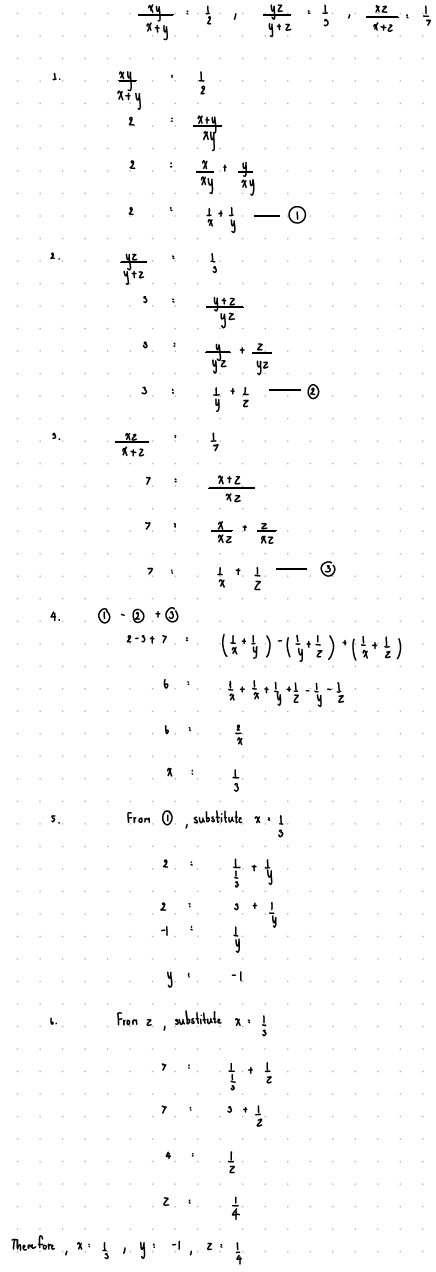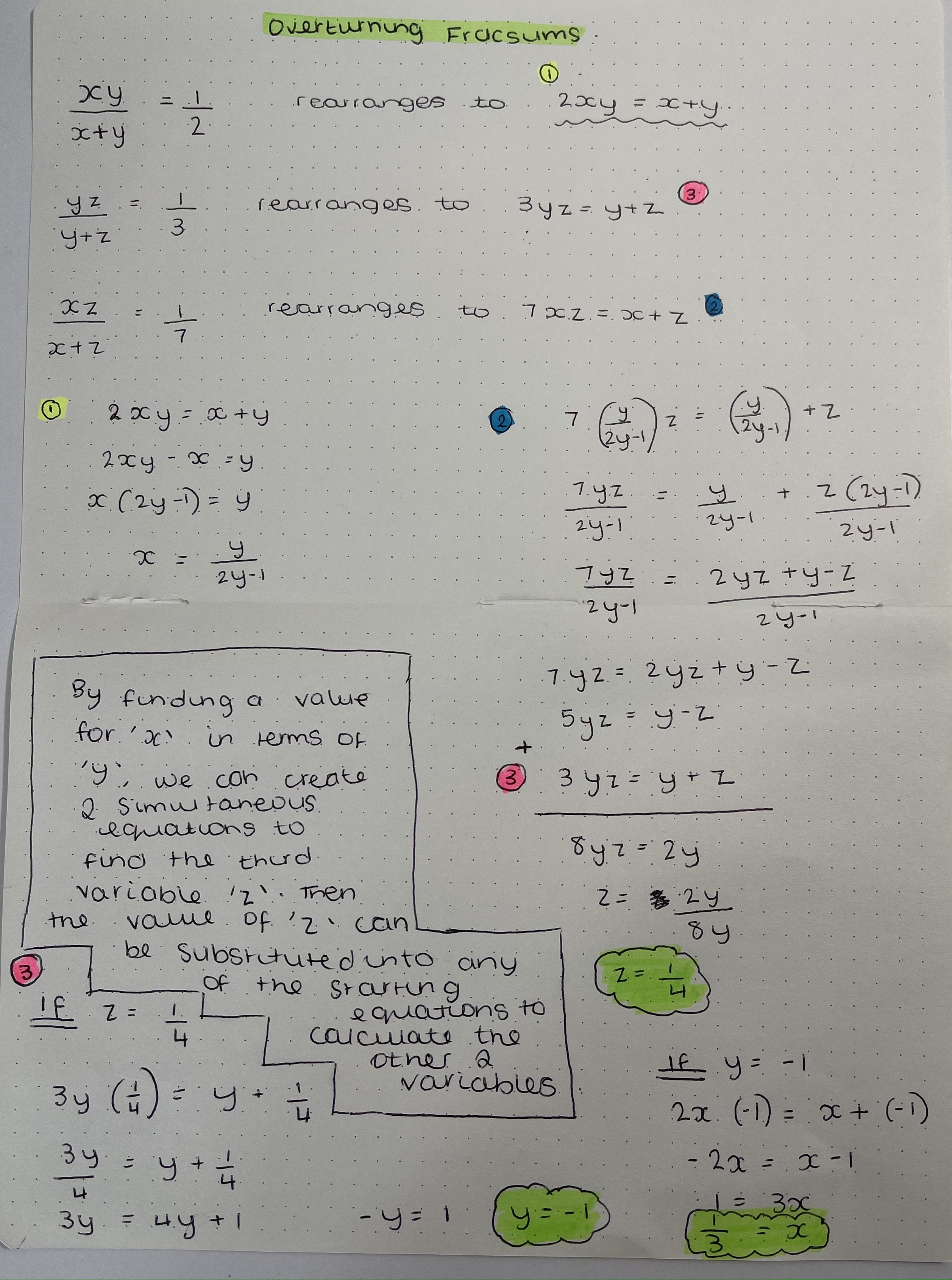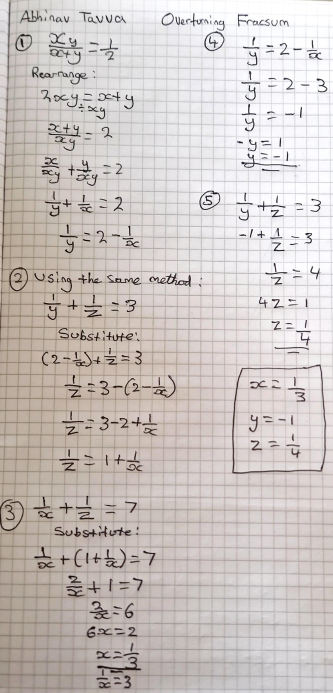#### You may also like### Real(ly) Numbers

If x, y and z are real numbers such that: x + y + z = 5 and xy + yz + zx = 3. What is the largest value that any of the numbers can have?### Pair Squares

The sum of any two of the numbers 2, 34 and 47 is a perfect square. Choose three square numbers and find sets of three integers with this property. Generalise to four integers.### Escriptions

For any right-angled triangle find the radii of the three escribed circles touching the sides of the triangle externally.

# Overturning Fracsum

##### Age 16 to 18Challenge Level

Well done to Nishad from Thomas Estley Community College, Jettarin from Stephen Perse Foundation, Abhinav from St. Olaves Grammar School, Yuk-Chiu from Harrow School and Deeya from the UK and Kesav, who all sent in full and correct solutions to this problem.

Nishad and Kesav sent in very similar solutions that involved first rearranging the equations, and then solving by elimination. Click to see Nishad's work.

$$\frac{xy}{x+y}=\frac{1}{2} \Rightarrow \frac{x+y}{xy}=2$$
We can split that LHS to get
$$\frac{1}{x} + \frac{1}{y} = 2$$
Similarly
$$\frac{1}{y} + \frac{1}{z} = 3$$
$$\frac{1}{x} + \frac{1}{z} = 7$$
Subtracting the first equation from the second equation gives
$$\frac{1}{z} - \frac{1}{x} = 1$$
Then adding this to the last equation gives
$$\frac{2}{z} = 8 \Rightarrow \frac{1}{z} = 4 \Rightarrow z = \frac{1}{4}$$
Hence
$$\frac{1}{x} + 4 = 7 \Rightarrow \frac{1}{x} = 3 \Rightarrow x = \frac{1}{3}$$
And finally
$$\frac{1}{y} = -1 \Rightarrow y = -1$$

Jettarin and Yuk-Chiu began in a very similar way, but combined the equations very neatly at the end. This is Jettarin's work (click to enlarge).Deeya solved the equations by using substitution:Abhinav also used substitution, but in a different form (click to enlarge):